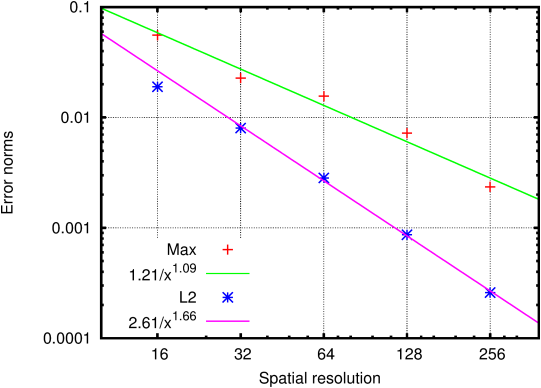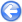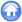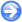### 13.6PASS: Creeping Couette flow between eccentric cylinders

Author
Stéphane Popinet
Command
sh wannier.sh wannier.gfs
Version
111108
Required files
wannier.c wannier.sh error.ref streamlines.gfv
Running time
1 minutes 32 seconds

This test case is similar to 6.3 but with eccentric cylinders. While the concentric cylinders case can be reduced to a one-dimensional equation in polar coordinates (the radial velocity component vanishes), this is not the case for eccentric cylinders. For this problem (also known as "journal bearing" flow), an exact analytical solution in the limit of Stokes flows was obtained by Wannier using conformal mapping .

The result of a computation using embedded solid boundaries is illustrated in Figure 179. The inner cylinder is rotating while the outer cylinder is fixed. Note the weak recirculation in the top part of the gap.

Figure 180 illustrates the rate of convergence with spatial resolution toward the analytical solution of Wannier.

 Figure 179: Streamlines for Stokes flow between eccentric cylinders.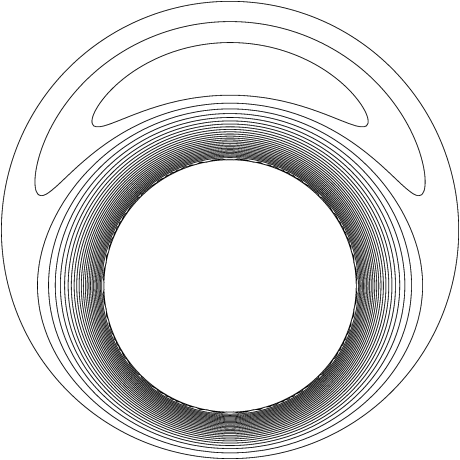Figure 180: Convergence of the maximum and RMS errors on velocity magnitude toward the analytical solution.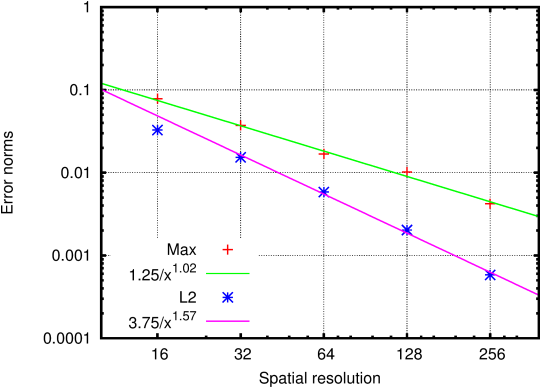#### 13.6.1PASS: Flow between eccentric cylinders using bipolar coordinates

Author
Stéphane Popinet
Command
sh bipolar.sh bipolar.gfs
Version
111108
Required files
bipolar.sh error.ref mesh.gfv
Running time
2 minutes 54 seconds

Same case as before but using bipolar coordinates to map the rectangular computational domain to the circular boundaries of the physical domain.

With these coordinates, solid embedded boundaries are not required and the number of grid points across the gap is constant (Figure 181). This markedly improves the convergence rate compared to the case using embedded solid boundaries (Figure 182).

Note also that this convergence can only be obtained when including the non-trivial viscous metric terms induced by the coordinate transformation.

 Figure 181: Bipolar computational mesh for Stokes flow between eccentric cylinders.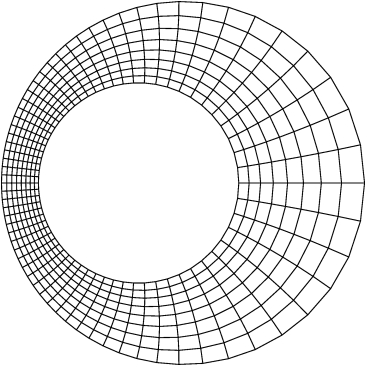Figure 182: Convergence of the maximum and RMS errors on velocity magnitude toward the analytical solution.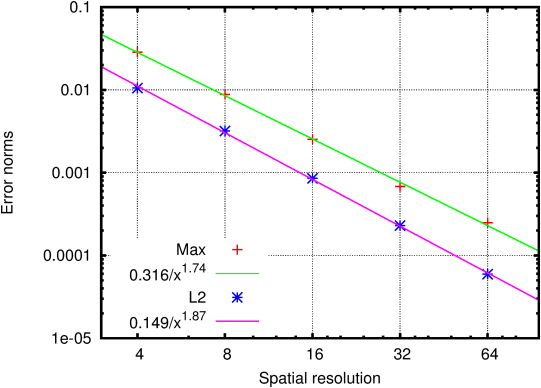#### 13.6.2PASS: Flow between eccentric cylinders on a stretched grid

Author
Stéphane Popinet
Command
sh ../wannier.sh stretch.gfs
Version
120229
Required files